## RS Aggarwal Class 8 Solutions Chapter 18 Area of a Trapezium and a Polygon Ex 18C

These Solutions are part of RS Aggarwal Solutions Class 8. Here we have given RS Aggarwal Solutions Class 8 Chapter 18 Area of a Trapezium and a Polygon Ex 18C.

Other Exercises

Tick the correct answer in each of the following :

Question 1.
Solution:
Parallel sides 14 cm and 18 cm
Distance between parallel sides (h) = 9cm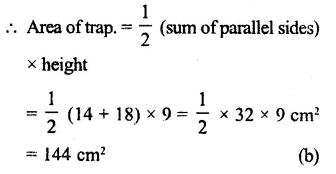Question 2.
Solution:
Length of parallel sides are 19 cm and 13 cm
Area of trapezium = 128 cm²
Distance between then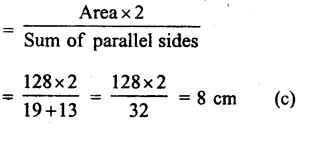Question 3.
Solution:
Ratio in parallel sides = 3:4
Perpendicular distance (h) = 12 cm
Area of trapezium = 630 cm²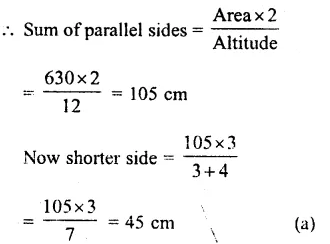Question 4.
Solution:
Area of trapezium = 180 cm²
and height (h) = 9 cm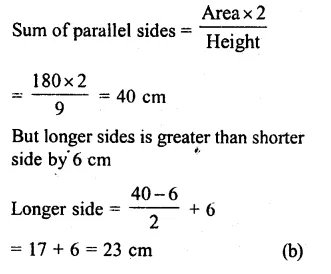Question 5.
Solution:
In the figure, AB || DC, DA ⊥ AB
DC = 7 cm, BC = 10 cm, AB = 13 cm
CL ⊥ AB
AD = DC = 7 cm
and LB – 13 – 7 = 6 cm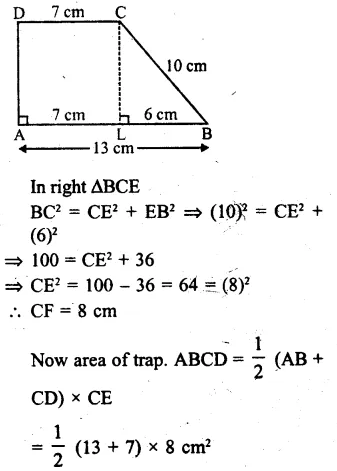Hope given RS Aggarwal Solutions Class 8 Chapter 18 Area of a Trapezium and a Polygon Ex 18C are helpful to complete your math homework.

If you have any doubts, please comment below. Learn Insta try to provide online math tutoring for you.